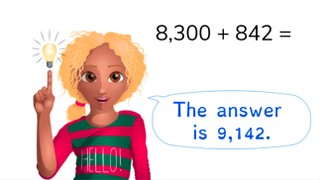# Addition to 10,000 with regrouping

Students learn to add to 10,000 with regrouping.8,000 schools use Gynzy92,000 teachers use Gynzy1,600,000 students use Gynzy

## General

Students learn addition to 10,000 with regrouping.

## Standards

CCSS.Math.Content.4.NBT.B.4

## Learning objective

Students will be able to add to 10,000 by regrouping the thousands, hundreds, or tens values.

## Introduction

Have the students solve the addition problems with simple numbers. The animals can be dragged away to reveal the answer.

## Instruction

Briefly go over the concepts of thousands numbers, hundreds numbers, tens numbers and ones values. First count how many blocks you have. Then count how many blocks are added on. Explain that you begin by adding the thousands numbers together. Then you add the hundreds numbers, tens numbers, and ones numbers together. Then you add all the numbers together to get the final answer. Now show that for the same problem, you can choose to split up numbers to solve in steps by regrouping. In this way, you can solve the problem by splitting the tens numbers into 50 and 20 and the ones values into 2 and 6. Practice the next problem together with the students. Ask the students which method they used to solve the problem. Have the students solve the next two problems on their own. Show the sum 3,850 + 468. Show that the girl adds the hundreds numbers, tens numbers and ones values. Then she adds everything together to get the answer. Now show the boy. He splits the second number up into hundreds numbers, tens numbers and ones values and adds them up in steps to get to 3,850. Have the students solve the next three problems on their own. For the story problem you can discuss the different methods that can be used to solve the problem. The students can solve the next two story problems on their own.

Check whether the students can add to 10,000 by regrouping with the following question:
- Which method do you use to add to 10,000 with regrouping?

## Quiz

The students first practice adding to 10,000 with regrouping using a visual aid. For the next assignment they just get a number problem. The third assignment is a story problem.

## Closing

Discuss the importance of being able to add to 10,000 with regrouping with the students. As a closing activity you can have the students form pairs or groups to solve the bowling score after two throws. Have them solve throw 1 and throw 2 and then add the answers together to get the final score.

## Teaching tips

Students that have difficulty adding to 10,000 with regrouping can first practice adding to 10,000 without regrouping using simple numbers. Then practice regrouping with simple numbers. Remind the students how you can split up numbers in steps, so that you can first fill in the number and then add up the rest.

## Instruction materials

MAB blocks

### The online teaching platform for interactive whiteboards and displays in schools

• Save time building lessons

• Manage the classroom more efficiently

• Increase student engagement